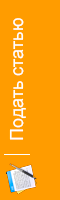Purpose of preventing interference – by giving temporarily code from one place into border, each subscriber has possibility communicating information and receiving signal of the six same frequencies.

Отрасль науки: Технические науки

B.O. Tuychiev1, Z.M. Ochilova2M.Sh. Choriyev3

1Tashkent University of information technologies Karshi branch,

Karshi, Uzbekistan

Senior Lecture, bekzod2702@mail.ru

2,3Tashkent University of information technologies Karshi branch,

Karshi, Uzbekistan

Student

MIMO TECHNOLOGY FOR MAXIMUM COVERAGE AREA OF 6X6 TO EXAMINE THE CALCULATION METHOD OF INPUT AND OUTPUT CHANNELS

Introduction

Up to now in mobile connection system to produce maximal border surround sphere, was used some radiofrequencies. Then was used different frequencies.  MIMO (Multiple input multiple output) makes possibility stay in some places, we can use same radiofrequencies. In order to interference use MIMO. MIMO has possibility to cover surround sphere 100% and also we can figure 6x6 wireless communication system characteristics.

To derive the channel characteristics, MIMO system transmits specified and known training signals regularly from all transmitters of the system and these transmitted signals are received at the receiver. Based on the received signals, the receiver calculates the characteristics of all channel paths from each transmitted antenna to each receiving antenna. In order to prove that MIMO work, the transmitted signal X has to be solved from the group of equations in equation (1) below. We also assume that the system is noise free and line of sight (LOS). Reference to figure 3 below, if the transmitted signal is represented to be X and the received signal Y. If the channel characteristics matrix is  , we may write

Y= (1)

If the channel matrix has N rows as many as there are transmitting antennas with index i. Then transmitted signal vector is written as X=  (2)

Also if the channel matrix has M columns, as there are receiving antennas with index j. Then the received signal vector is

Y=            (3)

These vectors are extended later to matrixes by inserting K samples into each column. The channel matrix contains path characteristics  as

Given Y=  then

(4)

The solution of X can deduce from equation (4) as

(5)

(6)

(7)

(8)

(9)

(10)

This implies that from equation (5)

(11)

Substituting equation (11) into equation (6), we have

(12)

(13)

(14)

(15)

(16)

(17)

Hence the solution for the transmitted signal X from equation (4) is given in a vector form as

Figure1. Channel characteristic of a 6x6 MIMO wireless communication system

Conclusion

Purpose of preventing interference – by giving temporarily code from one place into border, each subscriber has possibility communicating information and receiving signal of the six same frequencies.

Results of figuring in other words , , , ,  and , , , , ,   , communicating channel  between antennas and users, to distinguish some signals in the same frequencies serves by temporarily code.

Literatures

1. Multiple Input Multiple Output (MIMO) Operation Principles. Helsinki Metropolia University of Applied Sciences. Bachlor of Engineering Information technology, Thesis, 3 June 2013.
2. MIMO-OFDM Wireless Communications with Matlab.